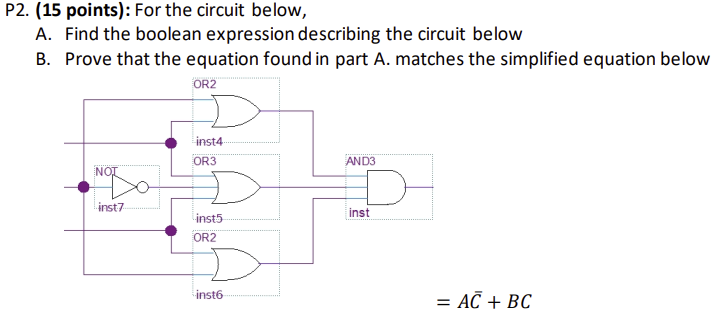Home / Expert Answers / Electrical Engineering / p2-15-points-for-the-circuit-below-a-find-the-boolean-expression-describing-the-circuit-belo-pa589

# (Solved): P2. (15 points): For the circuit below, A. Find the boolean expression describing the circuit belo ...P2. (15 points): For the circuit below, A. Find the boolean expression describing the circuit below B. Prove that the equation found in part A. matches the simplified equation below $1 \bar{C}+B C$

We have an Answer from Expert